# Duo mix

In one kilogram of meat of two colors is 650 g of pork, the rest is chicken. One kilogram of pork is 40 CZK more expensive than a kilogram of chicken. How many CZK cost one kilogram of chicken meat so that the price of one kilogram of meat of two colors is a maximum of 81 CZK.

k =  55 Kc/kg

### Step-by-step explanation: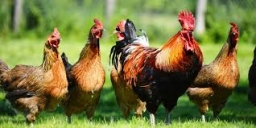We will be pleased if You send us any improvements to this math problem. Thank you!Tips to related online calculators
Do you have a system of equations and looking for calculator system of linear equations?
Do you want to convert mass units?

## Related math problems and questions:

• SweetsWe want to prepare 5 kg of sweets for 150 CZK. We will mix cheaper candy: 1 kg for 120 CZK and more expensive candy: 1 kg per 240 CZK. How much of these two types of candy is necessary to prepare this mixture?
• Coffee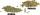Coffee merchant has coffee robusta and arabica species. 1 kg Robusta worth 450 CZK, Arabica 1 kg is 300 CZK more expensive. Calculate how many kilograms of Robusta and Arabica will need to produce 30 kg of the mixture so that the mixture cost is 490 CZK p
• Mixture of coffee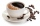They have to prepare a mixture of coffee in packing centres so that 1 kg costs 240 CZK. There are two types of coffee in stock at the price of 220 CZK per 1 kg and 300 CZK per 1 kg. How many kilograms of each species need to be mixed to prepare 50 kg of t
• CandiesThe price for 1 kg of more expensive candies is 125 CZK. The price for 1 kg of cheaper candy is 100 CZK. We mix two different mixtures of candy. A) The first mixture contains 2 kg more expensive and 0.5 kg cheaper candy. Calculate the price for 1 kg of th
• Tea mixture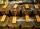Of the two sort of tea at a price of 180 CZK/kg and 240 CZK/kg we make a mixture 12 kg that should be prepared at a price of 200 CZK / kg. How many kilos of each sort of tea will we need to be mixed?
• CoffeeOne kilogram of cheaper coffee costs CZK 150, one kilogram of more expensive coffee costs CZK 200. We have to prepare a mixture of 35 kg of coffee for 180 CZK. How do we prepare the mixture (how many of which kind of coffee do we need)?
• Tea blendTea blends are maked from two kinds of tea. In standard tea mixture are two teas in the ratio 1:3 and 40 g costs 42 CZK. In the premium tea mixture are weighing two teas in the ratio 1:1 and 50 grams costs 60 CZK. How much cost 10 grams of more expensive
• Mixture of nutsThe mixture of nuts should be prepared from almonds, peanuts and cashew nuts ratio 1: 2: 3 (respectively). The price of almonds is 150 CZK/kg, the price of peanuts is 140 CZK/kg and the price of cashew nuts is 180 CZK/kg. The price of the mixture is deter
• BonbonsCreate a mixture of 50 kg of candy on price 700Kč. Candies has prices: 820Kč, 660Kč and 580Kč. Use cross rule.
• CandyThe price for 1kg of more expensive candy is 125 CZK. The price for 1kg of cheaper candy is 100 CZK. We make two different blends of candy. And now. The second blend contains 2kg of more expensive candy and several kg of cheaper candy. The price per 1 kg
• Two teas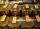A mixture weighing 10 kg was made from two types of tea. The price of tea was 160 CZK/kg and second tea 170 CZK/kg. The price of the mixture is 166 CZK/kg. How many kilograms of each type of tea had to be mixed?
• Bananas and mango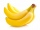5 kg of bananas and three kilograms of mango cost 146 CZK, two kilograms of bananas and 5 kilograms of mango cost 142 CZK. How much is a kilogram of bananas, and how many mangoes?
• Candies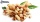In the confectionery, the price for 1 kg of pistachio candies cost CZK 360, and the price for 1 kg of hazelnut candies was CZK 280. Mixing these two types of sweets created a box of chocolates. How many grams of pistachios and how many grams of hazelnut c
• CandiesThere are two types of candies in the store at 20 CZK/kg and 80 CZK/kg. How much will be cost a mix if we mix 22kg cheaper and 4kg more expensive candies?
• Bonbons 2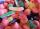Kilo sweets will cost 260 CZK. The first type has a price per 320 kg, the second type 240 CZK per kg. How many kilos of both kinds of sweets need to prepare a 35 kg mixture ?
• Czech coins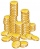John has 540 CZK, but he has 10 CZK coins and 20 CZK coins only, we knows that he has 10CZK coins 7times more that 20CZK coins, how many 10CZK coin and how many 20CZK coins he saved?
• MeatHalf a kilogram of pork and three-quarters of a kilogram of beef cost total 5.1 USD. One-quarter of a kilogram of beef and 1 kilogram of pork cost 4.9 USD. Find the price of 1 kg of each type of meat.